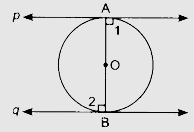# Prove that the tangents drawn at the ends of a diameter of a circle are parallel.

To do:

We have to prove that the tangents drawn at the ends of a diameter of a circle are parallel.

Solution:Let $AB$ be the diameter of a circle and $p$ and $q$ be the two tangents.

$OA \perp p$

$OB \perp q$

$\angle 1 = \angle 2 = 90^o$

This implies,

$p \| q$          (Two lines perpendicular to a line are parallel to each other)

Hence proved.